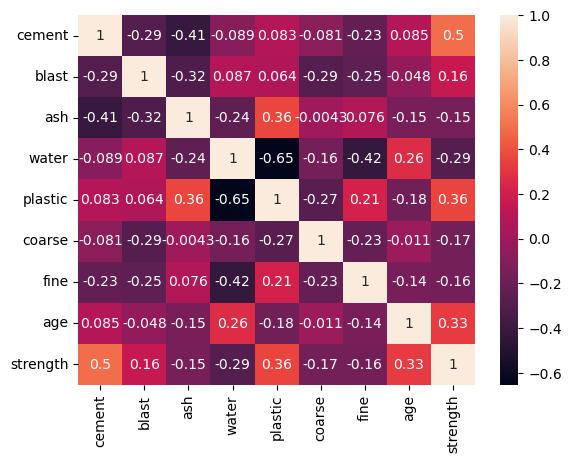top of page
Search

# Multivariate Data Analysis: Concrete data, 9 features, 1030 data

We have a dataset of 1030 data of concrete, downloaded from UCI repository https://archive.ics.uci.edu/dataset/165/concrete+compressive+strength . It collects 8 input features and 1 target feature:

• Cement: -- quantitative -- kg in a m3 mixture -- Input Variable

• Blast Furnace Slag -- quantitative -- kg in a m3 mixture -- Input Variable

• Fly Ash -- quantitative -- kg in a m3 mixture -- Input Variable

• Water -- quantitative -- kg in a m3 mixture -- Input Variable

• Superplasticizer -- quantitative -- kg in a m3 mixture -- Input Variable

• Coarse Aggregate -- quantitative -- kg in a m3 mixture -- Input Variable

• Fine Aggregate -- quantitative -- kg in a m3 mixture -- Input Variable

• Age -- quantitative -- Day (1~365) -- Input Variable

• Concrete compressive strength -- quantitative -- MPa -- Output Variable

The focus of this tutorial (Class of "Statistics and Machine Learning in Civil and Architectural Engineering", Aarhus University, Fall 2023) is Exploratory Data Analysis (EDA) of the dataset.

At first, we import the main libraries that we will use:

## IMPORT MODULES

1 import numpy as np 2 import pandas as pd 3 from OpenAIUQ import Stat as sta

In Lines 1-3 we import from OpenAIUQ the module Stat (prefix sta), numpy (prefix np), pandas (prefix pd)

## LOAD THE DATASET (1030 data of concrete)

1 df = pd.read_excel("Concrete_Data.xls") 2 dataset=np.array(df) 3 del df

In Line 1 we load the dataset (1030 data of concrete density), type=dataframe. Later we convert first a 2darray (Line2).

## EXPLORATORY DATA ANALYSIS

1 features = ['cement','blast','ash','water','plastic', 'coarse','fine','age','strength'] 2 concrete=sta.data2(dataset,features=features)

3 concrete.disp_summary(output='no')

4 concrete.summary

5 concrete.plot(pair='yes')

We convert the dataset (Lines 1-2) into the object concrete (type=data2 of Stat). The main statistical properties of the dataset are displayed through the method disp.summary (Lines 3-4). In Line 5 we build the figure pairplot of seaborn over the training data. The attribute concrete.summary and the figure concrete_pairplot provide a main picture of the training data.From concrete.summary it is seen that the concrete strength ranges from min=2.33 MPa to max=82.59 MPa. This implies that a good range of analysis for the strength can be x=[0, 90] MPa. The sample mean is m=35.81 Mpa, with coefficient of variation v=46.61% showing high degree degree of variability. It is not fully clear the reason of this great dispersion around the mean value. However, concrete_pairplot shows that the strength values have been measured:

• in different time slots ( shown from the data aligned vertically in the scatter plot age-strength)

• in different conditions, as shown from the histograms blast, ash, plastic (where there several zero entries in the features) and scatter plot blast-strength, ash-strength, plastic-strength.

Therefore the samples are not IID (independent and identically distributed). The sample skewness is g1=0.41 which implies some asymmetry toward the right tail, while the sample kurtosis g2=2.68 shows that extreme values are not likely.

## FEATURES

1 cement=concrete.d 2 blast=concrete.d 3 ash=concrete.d 4 water=concrete.d 5 plastic=concrete.d 6 coarse=concrete.d 7 fine=concrete.d 8 age=concrete.d 9 strength=concrete.d

In Lines 1-9 we build objects type=data1 from concrete data for more detailed statistical description of the features. It may be for example of interest to analyze the probabilistic distribution of the target.

1 edges=np.arange(0,95,5) 2 strength.plot_hist(bins=edges) 3 strength.ax.set_xticks(edges) 4 strength.ax.set_xlabel('strength [MPa]');

In Lines 1-4 we plot the histograms of the concrete strength, ranging from f=0 MPa to f=90 MPa, with binning size delta=5 MPa.1 strength.plot_cdf_emp()

2 strength.get_quantile(0.05) 3 print(strength.Q)

In Line 1 we plot the empirical cdf of the concrete strength. In Line 2 we evaluate the sample quantile 5% (characteristic value of the strength) which is f_k=10.97 MPa.## CORRELATIONS

1 for i in range(len(features)-1): 2 concrete.plot(x0=i,y0=8,regression='yes',hist='yes')

3 concrete.plot_corr(heatmap='yes',output='no') 4 concrete.R_df.round(3)

In Lines 1-2 we plot the jointplot of seaborn for each pair feature-target, including scatter plot, histograms and regression lines. The method concrete_plot is within a loop in order to consider the scatter plot between the strength and each input feature. Here x0=i means feature i (i.e. columns i+1) as x-axis, and y0=8 means feature 9 (i.e. strength)These plots are integrated with the values of the matrix of correlation (Lines 3-4).Although the coefficient of correlation defines only a linear relationship between target and input features, the trend can well be detected. It is seen so that the strength increase with the cement content, while decreases with the water content, as expected from the physics of the problem. High correlation values of the strength are with cement content (positive, +0.50), water (negative, -0.29). It appears that linear regression does not describe properly the correlation with age, since this is clearly a time-dependent phenomenon.

Concrete_Data
.xls

concrete
.ipynb